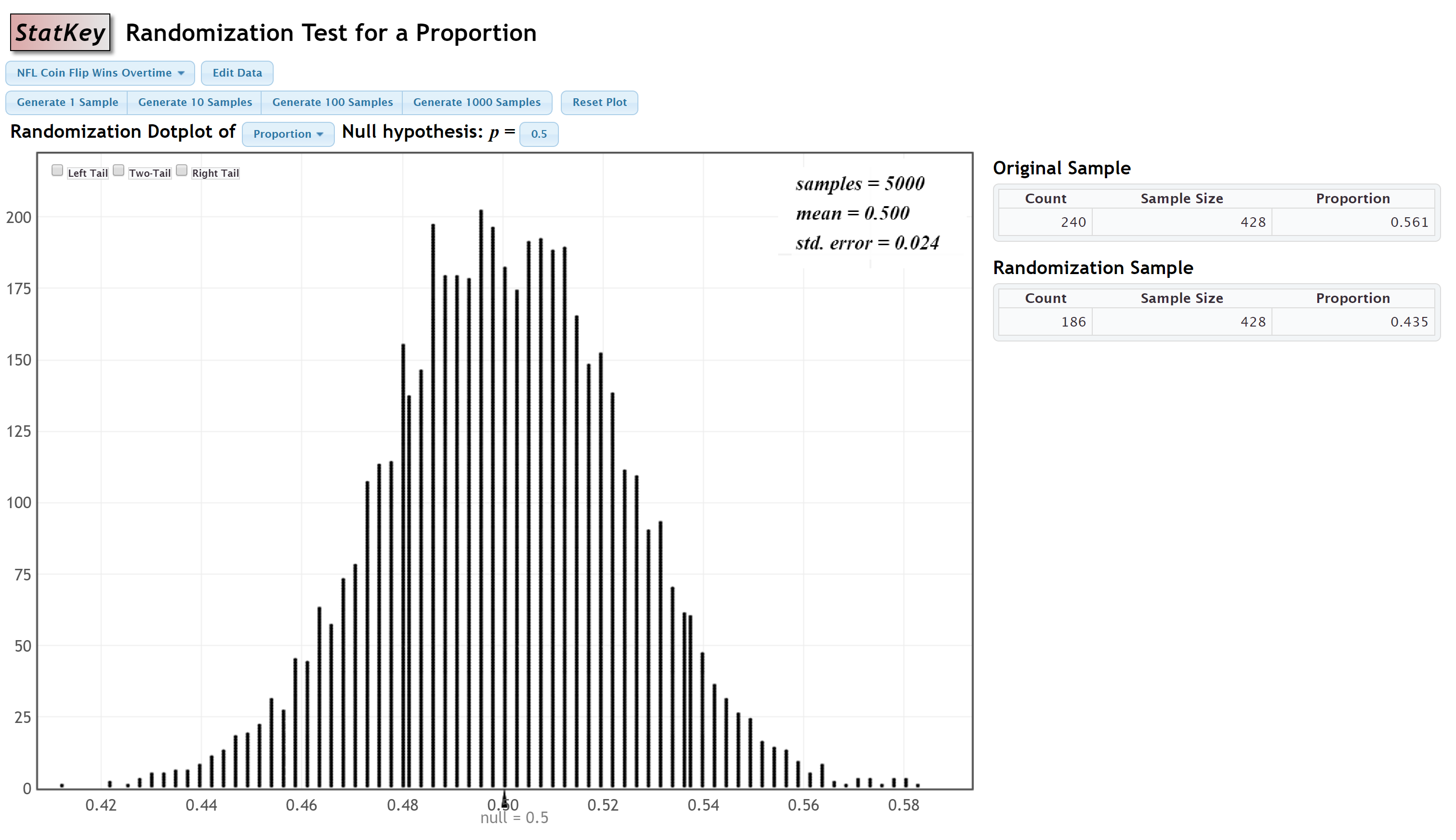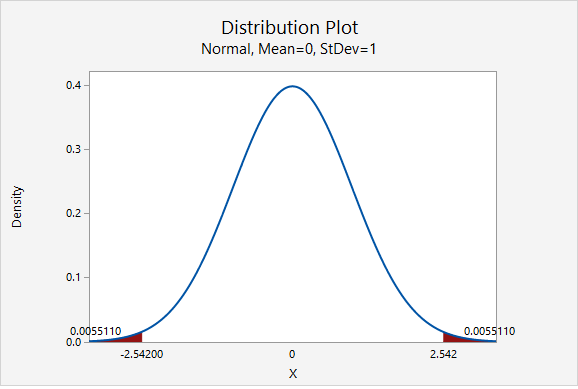# 7.4.1.3 - Example: Proportion NFL Coin Toss Wins

Research question: Is the proportion of NFL overtime coin tosses that are won different from 0.50?

StatKey was used to construct a randomization distribution:#### Step 1: Check assumptions and write hypotheses

From the given StatKey output, the sampling distribution is approximately normal.

$$H_0\colon p=0.50$$

$$H_a\colon p \ne 0.50$$

#### Step 2: Calculate the test statistic

$$test\;statistic=\dfrac{sample\;statistic-null\;parameter}{standard\;error}$$

The sample statistic is the proportion in the original sample, 0.561. The null parameter is 0.50. And, the standard error is 0.024.

$$test\;statistic=\dfrac{0.561-0.50}{0.024}=\dfrac{0.061}{0.024}=2.542$$

#### Step 3: Determine the p value

The p value will be the area on the z distribution that is more extreme than the test statistic of 2.542, in the direction of the alternative hypothesis. This is a two-tailed test:The p value is the area in the left and right tails combined: $$p=0.0055110+0.0055110=0.011022$$

#### Step 4: Make a decision

The p value (0.011022) is less than the standard 0.05 alpha level, therefore we reject the null hypothesis.

#### Step 5: State a "real world" conclusion

There is evidence that the proportion of all NFL overtime coin tosses that are won is different from 0.50